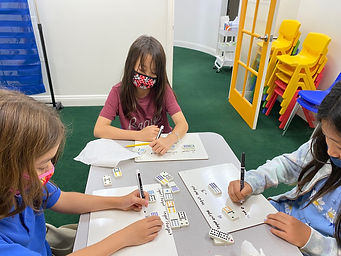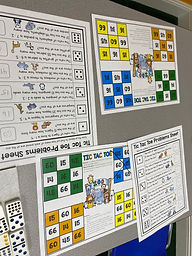## Ms. Dawn

### Target 1​

###### Lesson Type:

Continuation

Algebra

:

Function

Use input and output data to determine a function equation.

###### 1:

Understand that a function is a rule that gives one output to each input.

###### 2:

Understand that by studying the proportional relationship between inputs and outputs, a function (equation) can be derived.

4th

###### Vocabulary:

Input, Output, Function, Operation, Equation, Rule, Proportional

Activities:

1. Students created a rule for each dominoe they chose showing their understanding of input and output. They came up with different operation equations as their rules.### Home Exploration

###### Guiding Questions:## Absent Students:

### Target 2

:

###### 1:

Understand that a ratio represents a comparison of two quantities.

4th

###### Vocabulary:

Ratio, Meaning of ":" symbol, Meaning of word "To" in ratio terms, Quantities

Activities:

1. Students were given two sets of cards. One set had ratios using words and the other set had number ratios. Students needed to find the matching pair based on the words and number ratios.

2. Students played a game "Tic Tac Toe" based on solving ratio problems. Students rolled a die and needed to solve the problem of whatever number they rolled. First one to get Tic Tac Toe on any card won.

3. Students answered quesitons based on ratios through an interactive powerpoint. Students wrote their answers on an answer sheet. The questions asked in the powerpoint were geared to ratios.### Home Exploration

###### Guiding Questions:### Target 3

:

###### Vocabulary:

Activities:### Home Exploration